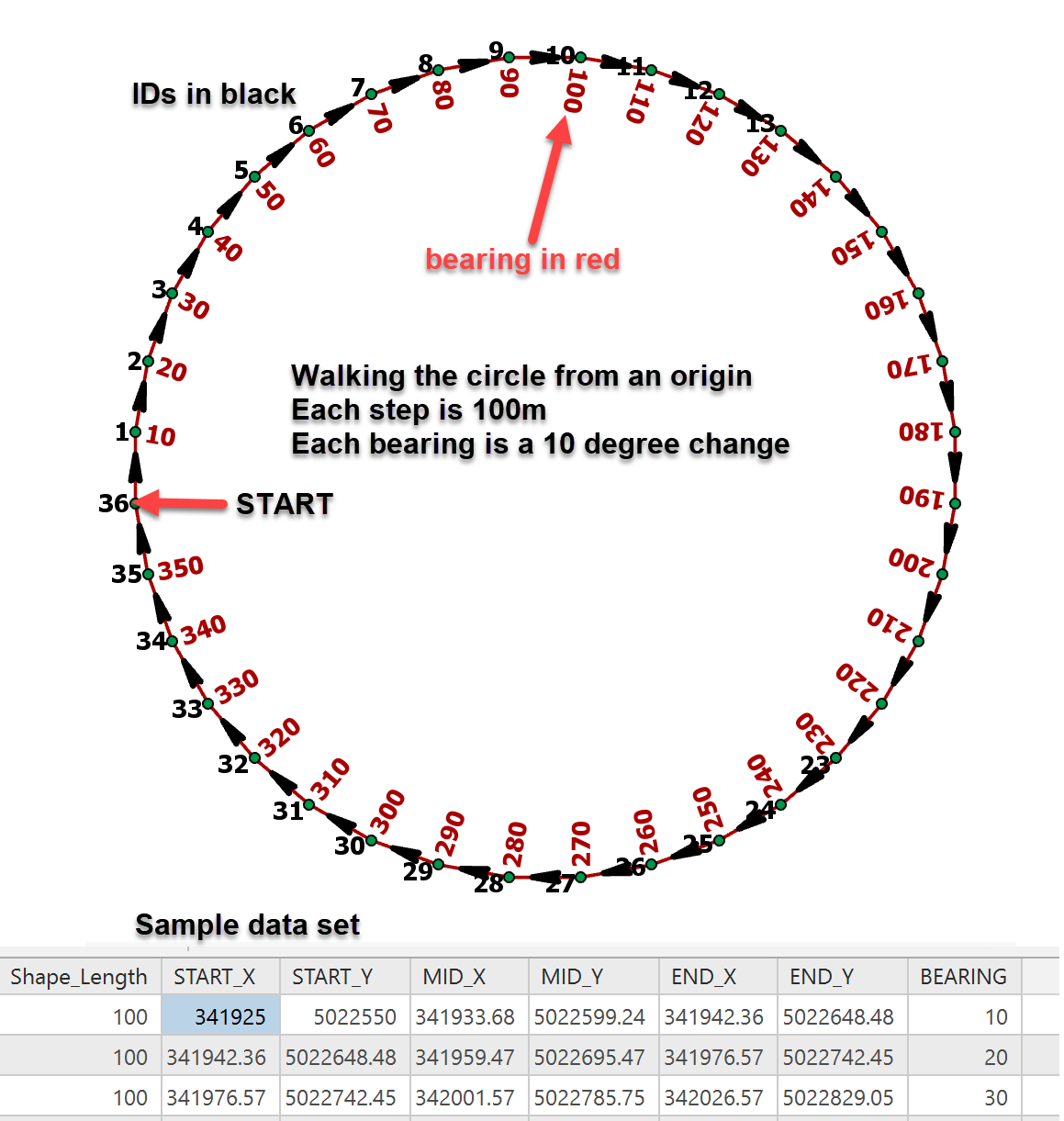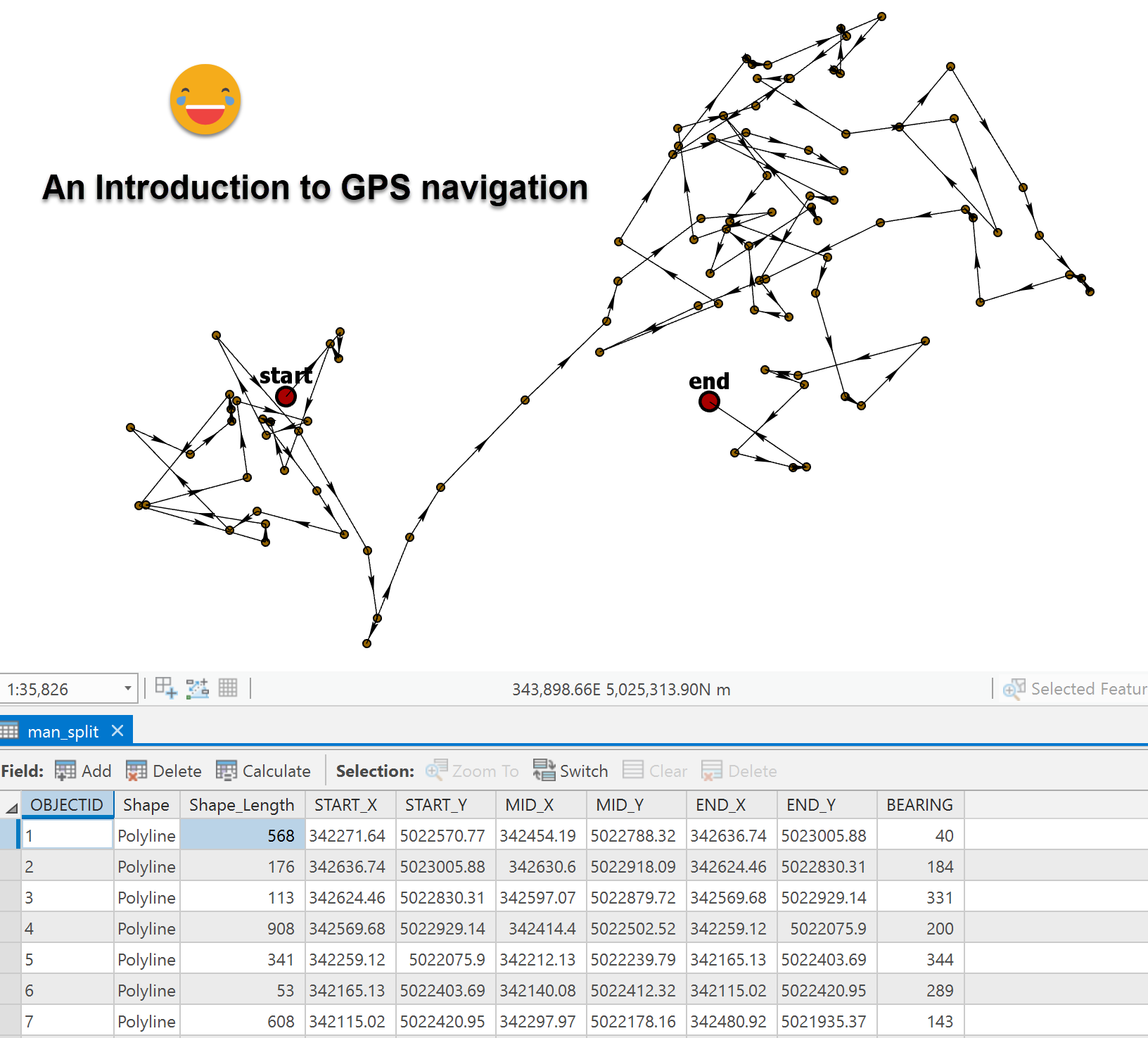# Origin, distances and bearings... geometry wanderings

660
0
01-20-2018 11:30 PM
Labels (1)byMVP Esteemed Contributor
2 0 660

Start at a point.  You are given a list of bearings and distances.  Where do you end up.

This comes up fairly often and the COGO tools allow you to create them from scratch.

Here is how you can do it using numpy and arcpy.

First a picture... given a location, you are instructed to move 100m in 10 degree increments between points.  You will obviously track a circle.  The traverse point locations can be constructed from base geometry.  The locations can then be saved to a featureclass.  The point featureclass can be converted to a continuous line, which can be segmented if you desire.  Total time, not much.The image above, shows the results of such a venture.

• I created my geometry using python/numpy
• I save the results to a featureclass using Numpyarraytofeatureclass (hence points)
• From those points I used Points to Line available at all license levels
• The continuous line was blown apart to segments using Split Lines at Vertices but alas, only available at the Advance level. .... ( I will save this obstruction to another blog, where I will show you how to do it with a standard licenseEDIT... From the second incarnation, you can produce a featureclass and use the XY to Line tool which is available at all license levels)
• Add Geometry Attributes enabled me to obtain the coordinates of the start, middle and end locations of the line.  This could have been done during the construction of the geometry as well.

An how many of us have taught a class where this happens....More to come....

Oh yes... code... NOW  the origin needs to be given in projected coordinates (ie UTM, State Plane, Web Mercator etc) since the calculations are done in planar units.  See my Vincenty implementation if you want to work with geodesic calculations.

``def dist_bearing(orig=(0, 0), bearings=None, dists=None):    """point locations given distance and bearing    :Notes:  for sample calculations    :-----    : - bearings = np.arange(0, 361, 10.)    : - dists = np.random.randint(10, 500, 36) * 1.0    : - dists = np.ones((len(bearings),))    :   dists.fill(100.)    """    rads = np.deg2rad(bearings)    sines = np.sin(rads)    coses = np.cos(rads)    dx = sines * dists    dy = coses * dists    x_n = np.cumsum(dx) + orig    y_n = np.cumsum(dy) + orig    data = np.vstack((bearings, dists, dx, dy, x_n, y_n)).T    frmt = "Origin (0,0)\n" + "{:>10s}"*6    names = ["bearings", "dist", "dx", "dy", "Xn", "Yn"]    print(frmt.format(*names))    for i in data:        print(("{: 10.2f}"*6).format(*i))    return data‍‍‍‍‍‍‍‍‍‍‍‍‍‍‍‍‍‍‍‍‍‍‍‍‍‍‍‍‍‍‍‍‍‍‍‍‍‍‍‍‍‍‍‍‍‍``

Optionally create a structured array that you can produce a featureclass from using this incarnation.

``def dist_bearing(orig=(0, 0), bearings=None, dists=None, prn=False):    """point locations given distance and bearing    """    orig = np.array(orig)    rads = np.deg2rad(bearings)    dx = np.sin(rads) * dists    dy = np.cos(rads) * dists    x_t = np.cumsum(dx) + orig    y_t = np.cumsum(dy) + orig    xy_f = np.array(list(zip(x_t[:-1], y_t[:-1])))    xy_f = np.vstack((orig, xy_f))    stack = (xy_f[:, 0], xy_f[:, 1], x_t, y_t, dx, dy, dists, bearings)    data = np.vstack(stack).T    names = ['X_f', 'Y_f', "X_t", "Yt", "dx", "dy", "dist", "bearing"]    N = len(names)    if prn:  # ---- just print the results ----------------------------------        frmt = "Origin (0,0)\n" + "{:>10s}"*N        print(frmt.format(*names))        frmt = "{: 10.2f}"*N        for i in data:            print(frmt.format(*i))        return data    else:  # ---- produce a structured array from the output ----------------        kind = ["<f8"]*N        dt = list(zip(names, kind))        out = np.zeros((data.shape,), dtype=dt)        for i in range(N):            nm = names[i]            out[nm] = data[:, i]        return out# --- from the structured array, use NumPyArrayToFeatureclass‍‍‍‍‍‍‍‍‍‍‍‍‍‍‍‍‍‍‍‍‍‍‍‍‍‍‍‍‍‍‍‍``

Again... more later

About the Author
Retired Geomatics Instructor at Carleton University. I am a forum MVP and Moderator. Current interests focus on python-based integration in GIS. See... Py... blog, my GeoNet blog...
Labels